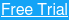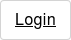# Price Rate of Change Indicator

The price rate of change indicator (PROC) or simply, rate of change indicator (ROC) is a price-based technical oscillator that is displayed in the sub-window. The PROC indicator is often referred to as purely a momentum oscillator.

As the name suggests, the PROC or ROC oscillator measures the rate of change in price, based on the look-back period. Based on the configurations used, the ROC indicator measures the current price with the price for the look-back period.

With some smoothing, the ROC oscillator moves around the 0-line from positive to negative. When momentum increases, the ROC oscillator moves from negative to above the 0-line and when momentum decreases, the ROC oscillator moves from positive and above the 0-line to negative.

As with all oscillators, the ROC can be used to time the market entry into a trend. It can also be used as a trend identification indicator as well as used to measure divergence in the momentum and price.

The first chart below shows the price rate of change oscillator applied to the daily chart. The setting for this indicator is the default 9-period setting.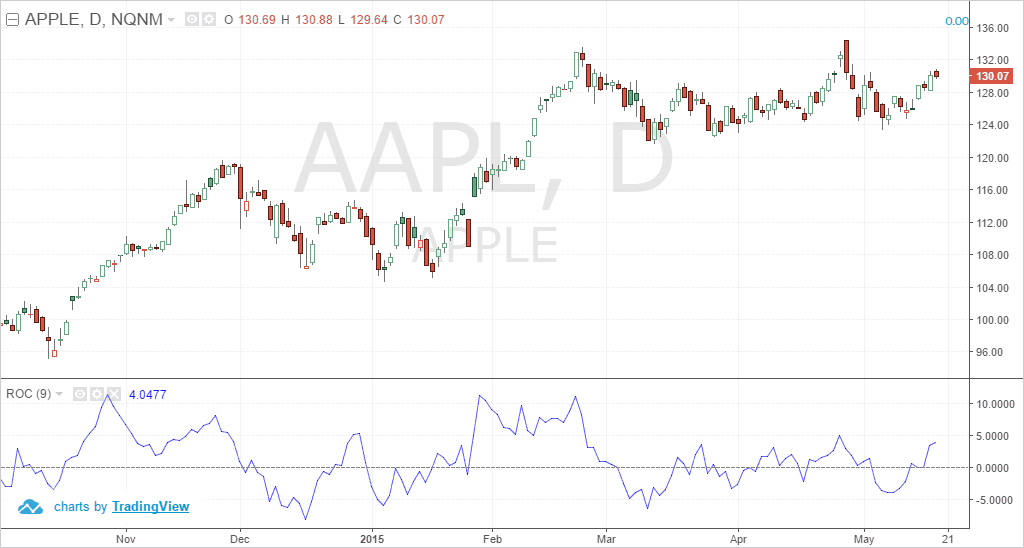Price Rate of Change Oscillator

As you can see in the above chart example, the PROC indicator moves within the fixed 0-line and indicates rising and falling momentum in price. This can be used as a technical oscillator to buy the dips in the uptrend or to sell the rallies in the downtrend.

The calculation of the PROC indicator is very simple and this technical oscillator is in fact very simple in its calculation.

## How to Calculate the Price Rate of Change Oscillator?

The calculation for the price rate of change oscillator is, as the name suggests, the rate of change. The calculation of the PROC indicator is defined below.

The ROC oscillator can also be plotted based on percentage terms instead of absolute values. For this calculation, the PROC indicator’s calculation is:

There are various settings that can be used for the ROC oscillator. For one, the common setting used for this indicator is 9-periods. Other commonly used indicator settings include 14 and 25 as well.

Readers should remember that there is no absolute number that can magically give the right settings. Rather, it is important to analyze the behavior of the security in order to use the right or the most appropriate settings for the ROC oscillator.

The volatility of the price of the asset being analyzed is of course of utmost importance. Using too small a number can lead to very choppy readings while using a higher configuration setting could potentially smooth the ROC to the point that signals can be very delayed.

For day traders, it is important to find a balance between the 9 and 14 periods setting for a daily chart.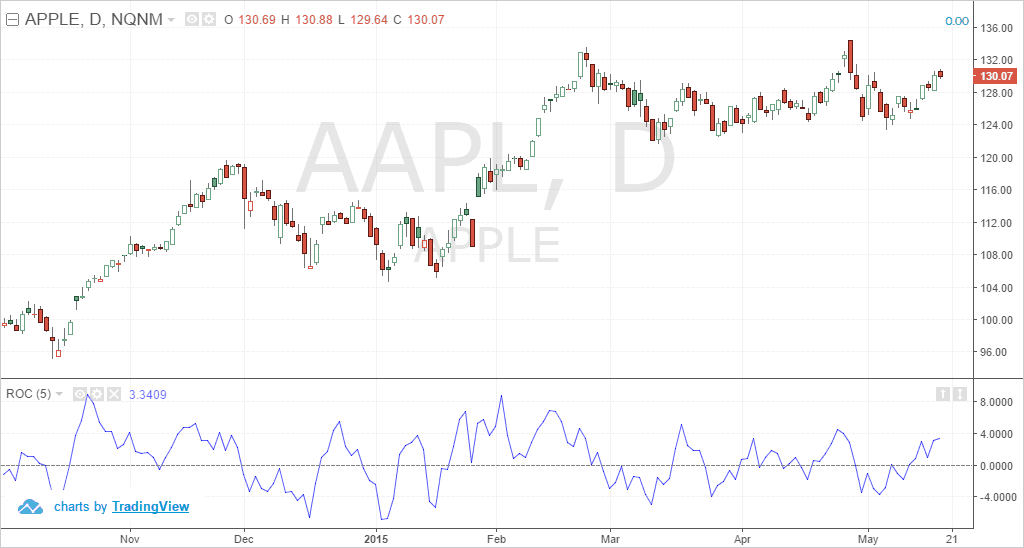Price Rate of Change Indicator Settings Configuration

In the above chart, you can see that when using a 5-period setting for the oscillator, the ROC can be choppy. While it might look easy in hindsight, it is important to note that the ROC oscillator will be difficult to pick up the tops and bottoms or in other words, rising and falling momentum in price.

Instead of simply applying the oscillator to the chart, traders should instead focus on analyzing the security properly before using the ROC indicator.

To illustrate the calculation with an example, let’s say that the price of an asset is at \$50 today. The price of the asset 9 days ago was \$40. Then, based on the ROC over the 9-period look-back the calculation would be ((50 – 40)/40) * 100 = 25.

## How can you use the rate of change oscillator in technical analysis?

The price rate of change oscillator can be used in a number of ways.

Here, we outline some of the methods involved mostly applicable for day trading or to time the price entry with the ROC indicator. The method of analysis with the price rate of change indicator is nearly the same as with most other commonly used oscillators such as the MACD for example.

### Using the ROC indicator as a timing tool

The ROC indicator can be used as timing tool within a trend analysis. We already know that price never moves in a straight line. This means that price posts a series of highs and lows within the trend.

For the trader, the challenge is of course in buying near the low point or the dip in the uptrend or selling near the high point or the rally in the downtrend. The ROC indicator can help traders to find these points. Of course, in order to know the trend, there are a number of ways.

Trends can be analyzed either by looking at the highs and lows or by looking at the signals from the technical indicators such as the moving averages.

In this first method of using the ROC to analyze the security, let’s look at a simple set up that involves moving averages and the ROC oscillator.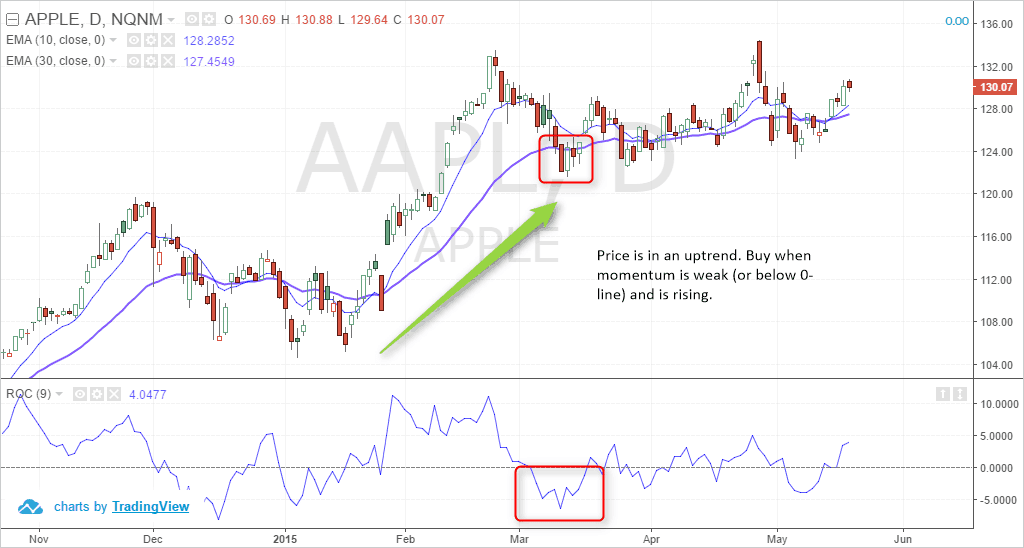ROC as a timing indicator in a trend

In the above example, we have a 10 and 30-period moving average applied to the daily chart. The ROC setting used here is 9-periods.

The concept is simple. Look to buy when momentum is at the weakest in the uptrend, or sell when momentum is starting to weaken. At the area marked by the square, you can see that the moving averages are aligned bullishly.

At this point, the ROC is also weaker but starting to rise. This suggests that price is likely to push higher. Additional confirmation can also be gotten from the candlestick patterns themselves. At the area highlighted, for example, we can notice an outside bar that was formed.

Other methods for validation can include looking at whether the fundamentals are bullish or not.

### Using the ROC for divergence

Divergence is common to all oscillators, regardless of what name they go by. The concepts of divergence are the same, whether you are using the Relative Strength Index (RSI), the Stochastics or the Price rate of change.

When the oscillator forms a fresh low but price does not, or when price forms a high but the oscillator does not, it can indicate a possible divergence and signal a correction.

Using this knowledge, traders can apply the price rate of change and look for divergence with price.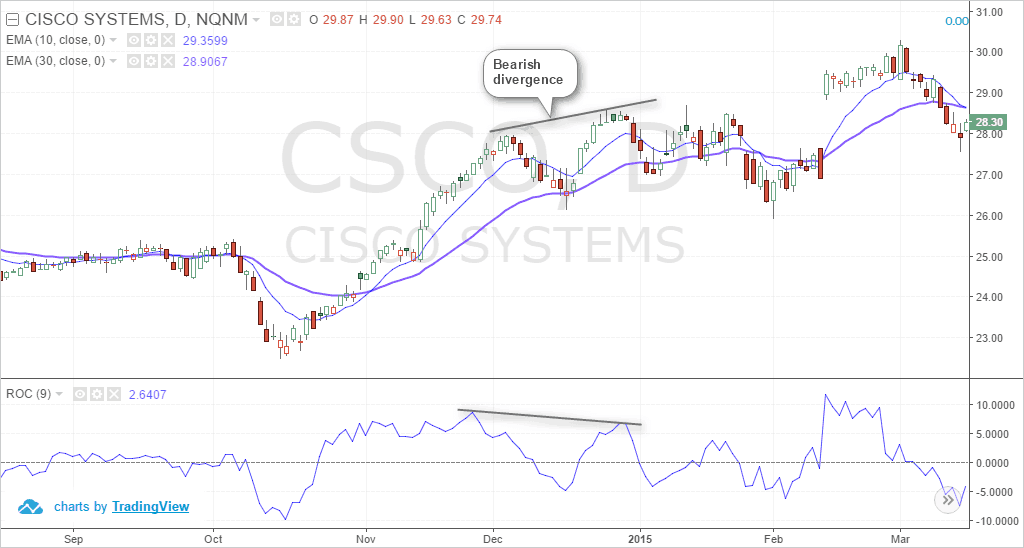Price Rate of Change Oscillator and Divergence

In the above example, we can see an instance of divergence that is forming. Here, price posted a higher high, but the ROC oscillator posted a lower high.

This divergence, known as bearish divergence can be used in two ways. A trader can either go short on the market after observing this phenomenon, or they can wait for the price correction to be completed and then enter long into the trend.

Other forms of divergence include the hidden bearish or bullish divergences, which are more powerful and can signal the trend continuation.

### The zero-line

The zero-line signal is another common method. Here, buy or sell signals can be taken based on when the ROC moves above or below the zero-line. Of course, using this method requires some experience as not all zero-line crossings are the same.

Sometimes, the ROC can dip below the zero-line only to reverse back higher. This is an important point to note. The ROC reacts to the price and not the other way around. Therefore, traders need to focus on the price and price action as well before trading this simple method with the ROC indicator.

Combining with trend indicators can be beneficial. This will help traders to ascertain the trend based on a moving average crossover and then validate this with the ROC.

This is illustrated in the next example below.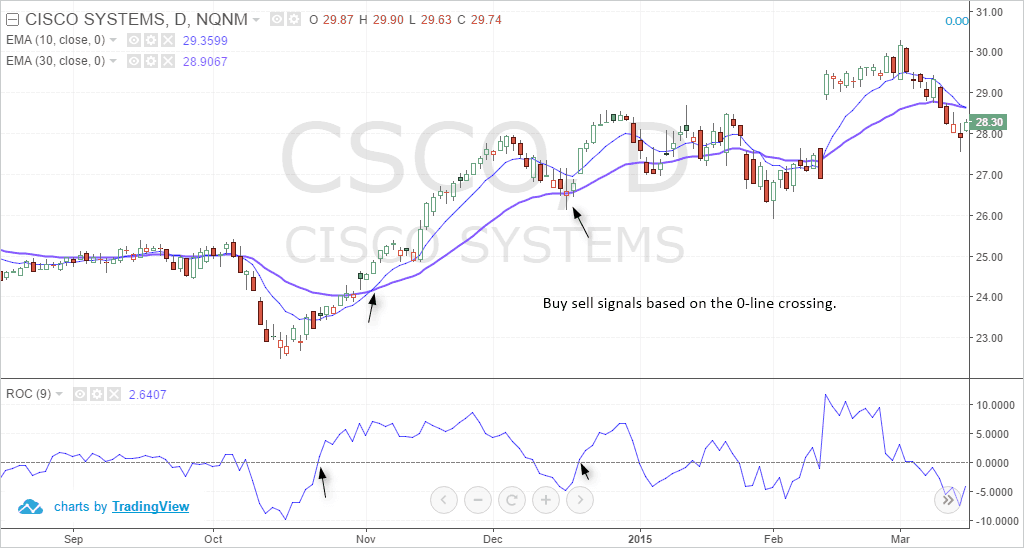Price Rate of Change zero-line crossing

In the above example, we look at how you can trade the price rate of change zero-line crossing.

In the first instance, we have the PROC indicator moving above the zero-line. This is a buy signal. However, notice that the moving averages were still bearish (consider the fact that moving averages are lagging, this will be a confirmation tool).

Once we have the 10 and 30 periods moving averages crossing over into bullish formation, we can then go long (because the PROC indicator is also above the zero-line). This suggests that the bullish momentum will continue.

In the second instance, while the moving averages were still bullish, the PROC indicator falls below the zero-line. This is a sell signal. But do you sell? No, because the trend is still bullish.

A few sessions later, we have the doji candlestick formation. This is also followed by the PROC indicator moving above the zero-line. In this case, the buy signal from the price rate of change indicator and the moving averages align together, signaling a long position in the market.

To trade with the zero-line crossing of the price rate of change indicator, you can use a number of other methods, including just price action to trade these signals successfully.

### Breakout trading with the ROC

Momentum oscillators such as the PROC indicator are very good at trading ranges and breakouts. This is because breakouts usually occur with strong momentum. It is common knowledge that not all breakouts are successful, and this is where a momentum oscillator, such as the PROC indicator, can help.

For breakout trading, one can use either the daily chart or an intraday chart with the appropriate settings for the ROC oscillator. However, one needs to find a balance so that the look-back period is not too choppy or not too smooth.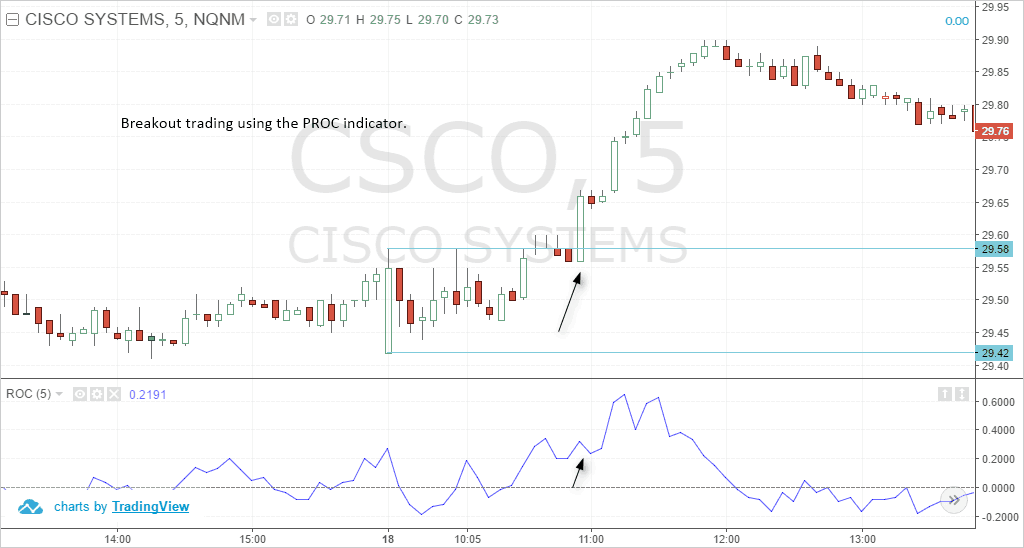Price rate of change indicator – Breakout trading

In the above example, we can see how price remained caught within a range of \$29.58 and \$29.42 on the 5-minute time frame. Here, we are using a 5-period look back from the ROC so that the momentum can be captured easily.

The basis for using the 5-period ROC setting is to capture the breakout and trade for a few dollars in profit.

Once price broke out strongly above \$29.58 resistance level or the upper range, the ROC signaled that it is above the zero-line. The trajectory is also rising higher and therefore, this suggests a continuation of the breakout.

Traders can simply go long after the close and set the stops to the low or the open of this bullish breakout candlestick. For profits, it is up to the trader to set the profits of \$2 – \$5 or a 1:2 or 1:3 risk rewards set up.

The above methods simply outline the many ways one can trade using the PROC or the ROC oscillator. There are certainly more methods of using the ROC than the ones outlined above.

## Which markets work best with the ROC oscillator?

There is no right answer to this. Some might argue that the rate of chance oscillator is ideally used with stocks. That may be true, but you can also use it with other markets including futures or even forex. The point to remember is that the ROC is merely a mathematical calculation.

For the ROC, it does not matter when the price of the security is a stock or futures or anything else. Therefore, you can use the price rate of change indicator in almost any market. The only main difference is that you need to focus on tweaking the oscillator to use the right settings.

The ROC is also independent of the time frame and merely looks at the sessions. So you can use the ROC on a daily chart as well as on a 5-minute chart. Futures day traders or even stock day traders can apply the rate of change oscillator to a chart and time frame of their choice and trade accordingly.

The downside with intra-day trading, especially stocks, is that the asset can become volatile. This is when traders need to pay attention to the technical indicator’s settings and adjust it accordingly. For example, if the first few hours are very volatile for the security, then it is best to use one setting, and when the volatile subsides, switch over to another setting.

In conclusion, the price rate of change oscillator is a momentum indicator and can be used to determine the short-term dips and rallies in price within a trend. The calculation of the PROC or the ROC is rather simple and does not require any complicated arithmetic.

This simplicity makes it easy for traders to make apply the ROC across different markets and timeframes.

## What is the Financial Stability Oversight Council (FSOC)?

The Financial Stability Oversight Council (FSOC) is the United States federal government...

## What is the Plunge Protection Team?

The Plunge Protection Team (PPT) is an informal term for the Working Group on Financial Markets. The working...